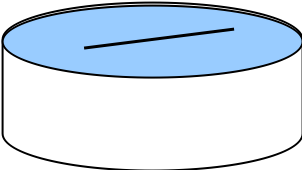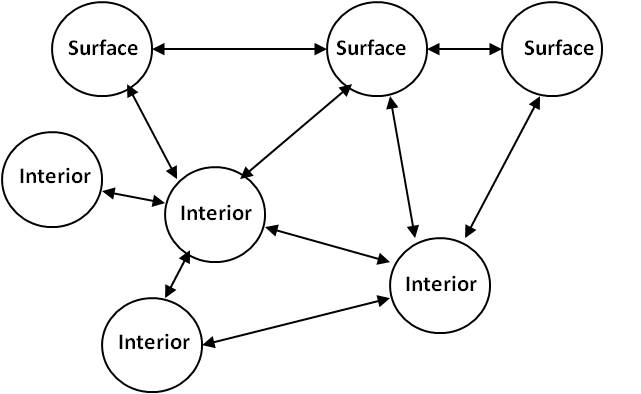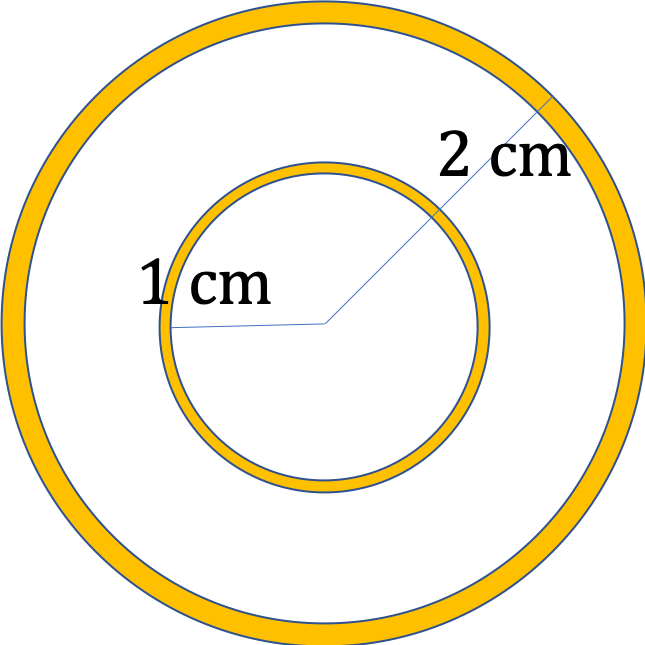## Section17.7Surface Tension

### Subsection17.7.1Surface Energy and Surface Tension

If you place a stainless steel needle carefully upon a calm surface of water, it will float even though the density of steel is considerably more than the density of water and the needle is a compact solid.

The needle floats not due to the buoyancy as you can easily demonstrate by placing the needle infinitesimally under the surface in which case the needle will sink. Instead, the needle floats because with gravity's pull, the needle stretches out the distance between the surface molecules, which push out on the needle, suporting its weight.A different behavior occurs at the surface due to the special situation of the surface molecules as compared to the molecules inside the volume.

A molecule inside the volume has other molecules of the fluid all around it so that, on average, there is no net intermolecular force on the molecules in the bulk. But, a molecule on the surface, is attracted by molecules of the liquid only on one side. This attractive force is balanced by the net upward impulse from movement of molecules below the surface.The balance of the attractive force and impulse of inner molecules determine the size and shape of the fluid surface. If you try to stretch the surface area, you would have to work against these attractive forces. This suggests that there is a potential energy stored per unit area of the surface.

Let $U$ denote the surface energy when the surface area is $A\text{,}$ and $U + \Delta U$ the surface energy when the surface area is $A+\Delta A\text{.}$ That is, $\Delta U$ be the energy cost when stretching the surface from area $A$ to the area $A+\Delta A\text{.}$ The rate at which surface energy changes is called surface tension, which we denote by the Greek letter “gamma”, $\gamma \text{.}$

\begin{equation} \gamma = \dfrac{\Delta U}{\Delta A}.\tag{17.7.1} \end{equation}

The units of surface tension will be $\text{J/m}^2\text{,}$ which is same as $\text{N/m}\text{.}$

Surface tension varies strongly with temperature. For instance, surface tension of water is $75.6\times 10^{-3}\text{N/m}$ at $0^{\circ}\text{C}\text{,}$ $72.8\times 10^{-3}\text{N/m}$ at $20^{\circ}\text{C}\text{,}$ and $58.8\times 10^{-3}\text{N/m}$ at $100^{\circ}\text{C}\text{.}$ This explains why hot water is better at cleaning than the cold water - with the reduced surface tension, water molecules can squeeze through microscopic spaces between fibers of the fabric, and remove any trapped dirt particles.

Similarly, soap lowers the surface tension of water. The detergent molecules, themselves may not be able to pass through the space between fabric fibers, but help water molecules to pass by lowering the surface tension of the mixture.

(Calculus)

Taking $\Delta A \rightarrow 0$ limit will give us the rate at which surface energy changes with area. Thus, we define surface tension by

\begin{equation*} \gamma = \lim_{\Delta A \rightarrow 0}\, \dfrac{\Delta U}{\Delta A}. \end{equation*}

### Subsection17.7.2Surface Tension in Stretching a Planar Film

As a simple yet powerful example of a surface tension application, consider a thin film of soapy water produced on a wire frame where one end is movable as shown in Figure 17.7.1.Figure 17.7.1. Stretching of a soap film. A constant force $F$ stretches the film by a length $\Delta x\text{,}$ increasing the surface energy by $\Delta U = F \Delta x$ and the surface area by $\Delta A = 2w\Delta x\text{,}$ where factor $2$ is due to top and bottom surfaces.

The force needed to move the wire outward by a distance $\Delta x$ depends on the width $w$ of the film. Furthermore, there are two sides of the film that need to be stretched together. Surface energy will increase due to the work done by the applied force F.

\begin{equation*} \Delta U = F\Delta x. \end{equation*}

The film has two surfaces, the top and the bottom. Therefore, the amount of increase in area would be twice the area on one side.

\begin{equation*} \Delta A = 2\times w\Delta x. \end{equation*}

Therefore, the surface tension will be

\begin{equation*} \gamma = \frac{\Delta U}{\Delta A} = \frac{F}{2w}. \end{equation*}

Assuming surface tension to be independent of the size of the bubble, find the energy stored in the surface energy of a spherical water droplet of $1\text{-mm}$ radius at $20^{\circ}\text{C}\text{.}$

Hint

Only one surface in a water droplet.

$9.1\times 10^{-7}\textrm{J}$

Solution

Let $R$ be the radius of the bubble. Since surface tension $\gamma$ is independent of the size of the bubble, we get

\begin{equation*} \Delta U = \gamma \Delta A. \end{equation*}

The surface area of the bubble is $4\pi R^2\text{.}$ Therefore, the bubble has surface energy

\begin{equation*} U(R) = 4\pi R^2 \gamma . \end{equation*}

In the droplet there is only one surface as opposed to the soap film on a wire frame where there were two surfaces. Putting the numerical values we obtain.

\begin{equation*} U(R) = 4\pi \times (10^{-3}\textrm{m})^2 \times (72.8\times 10^{-3}\textrm{N/m}) = 9.1\times 10^{-7}\textrm{J}. \end{equation*}

A rectangular film of mercury has a length of $2.0\text{ cm}$ and width $1.0\text{ cm}\text{.}$ It is stretched by $1.5\text{ mm}$ along its length while keeping the width same. What is the increase in surface energy of the film?

Data: $\gamma_\text{Hg} = 0.480\text{ N/m}\text{.}$

Hint

Use $\Delta U = \gamma\, \Delta A\text{.}$

$1.44\times 10^{-5}\text{ J}.$

Solution

From the definition of surface tension, the change in surface energy is given by

\begin{equation*} \Delta U = \gamma \Delta A. \end{equation*}

Here the change in area is for the two surfaces, above and below the film. Thus,

\begin{align*} \Delta A \amp = 2\times 1.5\times 10^{-3}\text{ m} \times 1.0\times 10^{-2}\text{ m}\\ \amp = 3.0 \times 10^{-5}\ \text{m}^2. \end{align*}

Therefore, the change in the surface energy will be

\begin{align*} \Delta U \amp = 0.480\text{ N/m} \times 3.0 \times 10^{-5}\text{ m}^2 \\ \amp = 1.44\times 10^{-5}\text{ J}. \end{align*}

A perfectly spherical bubble of diameter $2.0\text{ cm}$ is blown with Olive oil aboard the International Space Station. How much work was done in expanding the diameter from $1.0\text{ cm}$ to $2.0\text{ cm}\text{?}$

Data: $\gamma_\text{olive oil} = 0.032\text{ N/m} \text{.}$Hint

Use the surface energy of one spherical surface, $U(R) = \gamma \times 4\pi R^2 \text{.}$

$2.4\times 10^{-4}\text{ J}.$

Solution

The surface energy of a spherical surface of radius $R$ of a substance of surface tension $\gamma$ will be

\begin{equation*} U(R) = \gamma \times 4\pi R^2. \end{equation*}

Therefore, the change in energy for expanding one surface will be

\begin{equation*} U(R_2) - U(R_1) = 4\pi \gamma\left( R_2^2 - R_1^2\right) \end{equation*}

The olive oil bubble, not the drop, has two surfaces one inside and the other outside since the bubble encloses air pocket. Therefore, the work required will be twice as much. (You may say what about the impact of thickness of the oil film? we assume that the radius of the bubble is much greater than the thickness and hence thickness can be ignored.)

\begin{equation*} W = 2\times 4\pi \gamma\left( R_2^2 - R_1^2\right). \end{equation*}

The values here are $\gamma = 0.032\text{ N/m}\text{,}$ $R_1 = 0.01$ m, and $R_2 = 0.02$ m giving

\begin{equation*} W = 2.4\times 10^{-4}\ \text{J}. \end{equation*}

A drop of oil of radius $R$ is broken into $N$ idential droplets. If surface tension is $\gamma\text{,}$ what is the change in surface energy?

Check answer: if $R=10^{-2}\text{ m}\text{,}$ $4\pi\gamma = 0.1\text{ N/m}\text{,}$ $N=10^6\text{,}$ answer is $10^{-3}\text{ J\text{.}$ (adapted from Indian JEE Advanced 2017.)

Hint

Use mass conservation criterion and formula for the surface energy of a drop.

$4\pi\gamma R^2 \left(N^{1/3} -1\right)\text{.}$

Solution

From the conservation of mass, we must have masses of droplets add up to the mass of the originl drop. Since density is same, we will just equate the volumes. From this we can get an expression for the radius $r$ of the droplets.

\begin{equation*} N \times \frac{4}{3}\pi r^3 = \frac{4}{3}\pi R^3. \end{equation*}

Therefore,

\begin{equation*} r = N^{-1/3}\, R. \end{equation*}

Now, surface energy in a drop is $4\pi \gamma r^2\text{.}$ Therefore, we get the net change in surface enrgy to be

\begin{align*} \Delta U \amp = N \times 4\pi \gamma r^2 - 4\pi \gamma R^2 \\ \amp = 4\pi \gamma R^2 \left( N \times N^{-2/3} - 1\right) = 4\pi \gamma R^2 \left(N^{1/3} -1\right) . \end{align*}

When water is filled carefully in a glass, one can fill it to a height $h$ above the rim of the glass due to the surface tension of water.

To calculate $h$ just before water starts flowing, model the shape of the water above the rim as a disc of thickness h having semicircular edges, as shown schematically in Figure 17.7.7.

When the pressure of water at the bottom of this disc exceeds what can be withstood due to the surface tension, the water surface breaks near the rim and water starts flowing from there. If the density of water, its surface tension and the acceleration due to gravity are $10^3\text{ kg m}^{−3}\text{,}$ $0.07\text{ N m}^{−1}$ and $10\text{ ms}^{−2}\text{,}$ respectively, what is the value of $h$ (in mm)? (From Indian JEE, 2020)

Hint

Balance the force from surface tension acting at the rim by the outward force from varying pressure of the fluid through the bulging surface.

$h=\sqrt{14}\text{ mm}=3.74\text{ mm}.\text{.}$

Solution

From the discription of the problem, we interpret that by balancing forces on the extra water above the rim will give us the necessary condition for the stability of the bulging water. Let's think of bulging water in the shape of a cap and balance force on an element of bottom part of size $R\Delta \phi\text{,}$ where $R$ is radius of the rim and $\Delta \phi$ is angle subtended at the center of the tube.

There are two forces on the element: (1) horizontal force due to surface tension and (2) horizontal component of the force by pressure in the fluid acting on semicircular shaped element of width $R\Delta \phi$ and radius $a=h/2$ as shown in Figure 17.7.8.

The force from surface tension will be horizontal at the rim, giving

\begin{equation*} F_{st,x} = \gamma R\Delta\phi. \end{equation*}

The force due to fluid pressure on the strip shown in Figure 17.7.8 can be computed by adding contributions from elements of te strip. An area element of the strip will be

\begin{equation*} \Delta A = R\Delta\phi \times a\Delta \theta. \end{equation*}

Then at coordinate $y$ where height of fluid above is $a-y\text{,}$ we get pressure $\rho g (a-y)\text{.}$ Therefore, force has magnitude $dF=\rho g (a-y)\Delta A\text{.}$ We want its horizzontal component to balance the horizontal force of tension. We have $dF_x$ to be

\begin{equation*} dF_x = dF\,\cos\theta = \rho g (a-y)R\Delta\phi \times a\Delta \theta\,\cos\theta. \end{equation*}

Summing over all elements alog strip is done by going to the infinitesimal limit in $\Delta \theta$ and integrating. Since $y=a\sin\theta\text{,}$ we need to include it inside integration.

\begin{align*} F_x \amp = \rho g R\Delta\phi \times a \, \int_{-\pi/2}^{\pi/2}(a-y)\cos\theta d\theta\\ \amp= \rho g R\Delta\phi \times a \times 2a \\ \amp= \rho g R\Delta\phi \times \frac{h^2}{2}. \end{align*}

Balancing this with the force of tension yields

\begin{equation*} \rho g R\Delta\phi \times \frac{h^2}{2} = \gamma R\Delta\phi. \end{equation*}

Simplifying this we get

\begin{equation*} h = \sqrt{\frac{2\gamma}{\rho g}}. \end{equation*}

Therefore,

\begin{equation*} h = \sqrt{\frac{2\times 0.07}{10^3\times 10}} = \sqrt{14}\,\times 10^{-3}\text{ m} = 3.74\text{ mm}. \end{equation*}

### Subsection17.7.3Capillary Action

When you dip a wide tube in a fluid, the level of the fluid in the tube is same inside the tube as outside.

However, when very thin tubes are dipped in a liquid, this is not the case. Instead, liquid rises to various heights depending on the diameter of the opening as illustrated in Figure 17.7.9. The effect is called the capillary action.

In Figure 17.7.9 we see that level of the fluid inside the tube may be above or below the level in the wider container depending upon the contact angle. In the fogure, the two center tubes have contact angle less than 90° and two tubes to the right two tubes have contact angle greater than 90°.

The meniscus may be convex, e.g., water in a glass tube, or convex, e.g., meercury in a glass tube as illustrated in Figure 17.7.10. The reason behind these shapes has to do with the comparative attraction between molecules of the fluid and between fluid and the wall material.

If fluid is more attracted to the material of the tube that to each other, then the meniscus will be concave, i.e., it will crrep up on the wall. If the fluid has higher attraction among themselves, then it will minimize energy by having a convex surface, i.e., it will curl up on itself away from the wall.

To understand the capilary action, let us an open tube of radius $r$ dipped in a fluid of density $\rho$ so that the fluid rises in the tube by a height $h$ above which there is a spherical meniscus of radius of curvature $R$ and contact angle $\theta$ as shown in Figure 17.7.11.

The pressure difference due to surface tension at the miniscus is given by Young-Laplace's equation to be

\begin{equation} P_0 - P_1 = \frac{2\gamma}{R} = \frac{2\gamma\,\cos\theta}{r}.\tag{17.7.2} \end{equation}

This pressure is pushing down on the fluid in the tube. From the figure, pressure difference in the liquid of height $h\text{,}$ pushing up on the fluid is

\begin{equation} P_0 - P_1 = \rho g h. \tag{17.7.3} \end{equation}

At equilibrium the two pressures will be balanced giving

\begin{equation} h = \frac{2\gamma\,\cos\theta}{ \rho g r}.\tag{17.7.4} \end{equation}

If the meniscus is flat, then $\theta=0\text{,}$ giving

\begin{equation*} h = \frac{2\gamma}{ \rho g r},\ \ \text{(flat meniscus)} \end{equation*}

We find that height $h$ is inversely proportional to the tube radius $r\text{.}$ If we use the surface tension of water at $20^{\circ}\text{C}$ in this relation, we find that water will rise $3\text{ cm}$ in a tube of inner diameter $1\text{ mm}\text{.}$

A liquid of density $2.0\text{ g/cc}$ rises by $1.8\text{ cm}$ in a capillary tube of radius $0.075\text{ cm}$ when dipped vertically in the liquid. What is its surface tension?

Hint

Use capillary action.

$0.132\text{ N/m}\text{.}$

Solution

Let us assume flat meniscus. From the capillary action formula $h = 2\gamma/ \rho g R\text{,}$ we get

\begin{equation*} \gamma = \dfrac{1}{2}\,\rho g R h. \end{equation*}

We can either convert all units in $\text{kg,m}$ or work in $\text{g,cm}\text{.}$ Let's convert the units.

\begin{align*} \rho \amp = 2.0\text{ g/cc} = 2000\text{ kg/m}^3\\ R \amp = 0.075\text{ cm} = 7.5\times 10^{-4}\text{ m}\\ h \amp = 1.8\text{ cm} = 1.8\times 10^{-2}\text{ m}\\ g \amp = 9.81\text{ m/s}^2 \end{align*}

Therefore,

\begin{equation*} \gamma = 0.132\text{ N/m}. \end{equation*}

Water rises to a height of $2.5\text{ cm}$ in a capillary tube of radius$0.50\text{ mm}\text{.}$ If surface tension of water at that temperature is $72 mN/m\text{,}$ what is the angle of contact?

Hint

Use capillary action formula.

$31.6^\circ\text{.}$

Solution

We will use the capillary action formula derived in this section.

\begin{equation*} h = \frac{2\gamma\,\cos\theta}{ \rho g r}. \end{equation*}

We use $\rho = 1000\,\text{kg/m}^3\text{.}$ We get

\begin{equation*} \cos\theta = \frac{1000\times 9.81 \times 0.5\times 10^{-3} \times 2.5\times 10^{-2} }{ 2\times 0.5\times 10^{-3} } = 0.85. \end{equation*}

This gives $\theta = 31.6^\circ\text{.}$